Rational Expressions: More Examples
(page 3 of 3)• Simplify the following:
•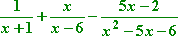To find the common denominator, I'll first have to factor the quadratic in the third denominator:

x2 – 5x – 6 = (x – 6)(x + 1)

Fortunately for me, the quadratic denominator didn't introduce any new factors to the problem, so the common denominator will be (x – 6)(x + 1).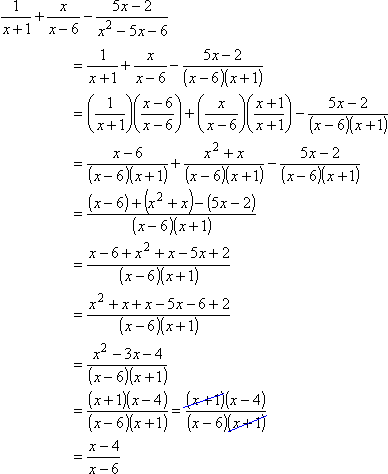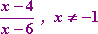You might not need to include the "for x not equal to –1" part of the solution.

• Simplify the following:
•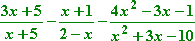First I'll factor the quadratic in the third denominator:

x2 + 3x – 10 = (x + 5)(x – 2)

Note that these factors almost match the other denominators, but the second fraction's denominator is "backwards". How can I fix that? I can fix it by remembering the following:

5 – 3 = 2
3 – 5 = –2

The point of these two subtractions is that, when I reversed the subtraction, I got the same answer except for the sign. So I can reverse the subtraction in the second fraction's denominator, as long as I remember to also reverse the sign. This is what that looks like: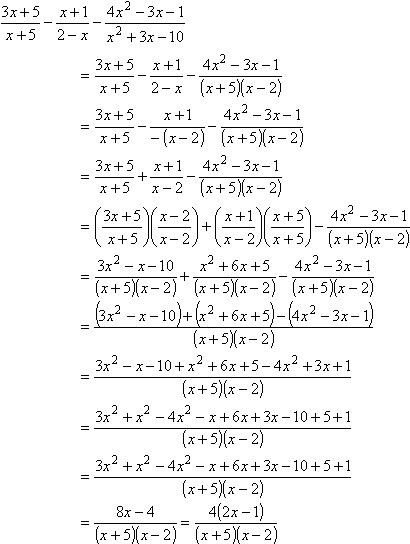I factored the numerator, but nothing cancels out. As you can see, I had to factor a denominator, multiply two of the fractions to get a common denominator, multiply those two fractions' numerators, add, simplify, and then factor again. You should expect to see some problems that are at least this involved. They're not as much "complicated" as they are "long and annoying". Work them out step-by-step as I did above, and you'll get the right answers fairly regularly. In this case, the answer is: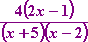When you're adding and subtracting rationals, don't try to do a lot of steps in your head, or skip steps or do half-steps (like leaving out the denominators in your calculations), or you'll pretty much guarantee yourself the wrong answer. Take the time to do every step completely and carefully as you "practice" on the homework, so you have a good chance of getting these exercises right on the test.

<< Previous  Top  |  1 | 2 | 3  |  Return to Index